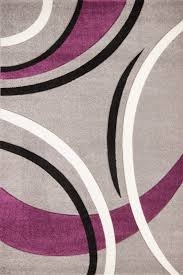# Flooring 46511

The carpet covers 8 m2 of flooring, which is one third of the entire floor area. How big is the whole floor?

x =  24 m2

### Step-by-step explanation:Did you find an error or inaccuracy? Feel free to write us. Thank you!

Tips for related online calculators
Need help calculating sum, simplifying, or multiplying fractions? Try our fraction calculator.
Do you have a linear equation or system of equations and looking for its solution? Or do you have a quadratic equation?

#### Grade of the word problem:

We encourage you to watch this tutorial video on this math problem: